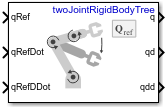# Joint Space Motion Model

Model rigid body tree motion given joint-space inputs

• Library:
• Robotics System Toolbox / Manipulator Algorithms## Description

The Joint Space Motion Model block models the closed-loop joint-space motion of a manipulator robot, specified as a `rigidBodyTree` object. The motion model behavior is defined by the `Motion Type` parameter.

## Ports

### Input

expand all

n-element vector representing the desired joint positions of radians, where n is the number of nonfixed joints in the `rigidBodyTree` object of the ```Rigid body tree``` parameter.

#### Dependencies

To enable this port, set the ```Motion Type``` parameter to `Computed Torque Control`, `PD Control`, or ```Independent Joint Motion```.

n-element vector representing the desired joint velocities of radians per second, where n is the number of nonfixed joints in the `rigidBodyTree` object of the ```Rigid body tree``` parameter.

#### Dependencies

To enable this port, set the ```Motion Type``` parameter to `Computed Torque Control`, or `Independent Joint Motion`.

n-element vector representing the desired joint velocities of radians per second squared, where n is the number of nonfixed joints in the `rigidBodyTree` object of the ```Rigid body tree``` parameter.

#### Dependencies

To enable this port, set the ```Motion Type``` parameter to `Computed Torque Control`, `PD Control`, or ```Independent Joint Motion```.

A 6-by-m matrix of external forces for the m bodies in the `rigidBodyTree` object of the ```Rigid body tree``` parameter.

#### Dependencies

To enable this port, set the ```Show external force input``` parameter to `on`.

### Output

expand all

Joint positions output as an n-element vector in radians or meters, where n is the number of nonfixed joints in the `rigidBodyTree` object of the ```Rigid body tree``` parameter.

Joint velocities output as an n-element vector in radians per second or meters per second, where n is the number of nonfixed joints in the `rigidBodyTree` object of the ```Rigid body tree``` parameter

Joint accelerations output as an n-element vector in radians per second squared or meters per second squared, where n is the number of nonfixed joints in the `rigidBodyTree` object of the ```Rigid body tree``` parameter

## Parameters

expand all

Robot model, specified as a `rigidBodyTree` object. You can also import a robot model from an URDF (Unified Robot Description Formation) file using `importrobot`.

The default robot model, `twoJointRigidBodyTree`, is a manipulator with revolute joints and two degrees of freedom.

Type of motion, specified as a string scalar or character vector that defines the closed-loop joint-space behavior that the object models. Options are:

Inputs to control the robot system. Options are:

#### Dependencies

To enable this parameter, set the ```Motion Type``` parameter to `Computed Torque Control` or `Independent Joint Motion`.

Damping ratio use to decay system oscillations. A value of `1` results in no damping, whereas `0` fully dampens the system.

#### Dependencies

To enable this parameter, set the ```Specification format``` parameter to ```Damping Ratio / Natural Frequency```.

Frequency of the system oscillations if unimpeded, specified in Hz.

#### Dependencies

To enable this parameter, set the ```Specification format``` parameter to ```Damping Ratio / Natural Frequency```.

The time taken for each joint to reach steady state, measured in seconds.

#### Dependencies

To enable this parameter, set the ```Specification format``` parameter to `Step Response`.

The maximum value that the system exceeds the target position.

#### Dependencies

To enable this parameter, set the ```Specification format``` parameter to `Step Response`.

Proportional gain for proportional-derivative (PD) control, specified as a scalar or n-by-n matrix, where n is the number of nonfixed joints in the `rigidBodyTree` object of the ```Rigid body tree``` parameter.

#### Dependencies

To enable this parameter, set the ```Specification format``` parameter to `PD Control`.

Derivative gain for proportional-derivative (PD) control, specified as a scalar or n-by-n matrix, where n is the number of nonfixed joints in the `rigidBodyTree` object of the ```Rigid body tree``` parameter

#### Dependencies

To enable this parameter, set the ```Specification format``` parameter to `PD Control`.

Enable this parameter to input external forces using the `FExt` port.

#### Dependencies

To enable this parameter, set the ```Motion Type``` parameter to `Computed Torque Control`, `PD Control`, or ```Open Loop Dynamics```.

Initial joint positions, specified as a n-element vector or scalar in radians. n is the number of nonfixed joints in the `rigidBodyTree` object in the ```Rigid body tree``` parameter.

Initial joint velocities, specified as a n-element vector or scalar in radians per second. n is the number of nonfixed joints in the `rigidBodyTree` object in the ```Rigid body tree``` parameter.

• `Interpreted execution` — Simulate model using the MATLAB® interpreter. For more information, see Simulation Modes (Simulink).

• `Code generation` — Simulate model using generated C code. The first time you run a simulation, Simulink® generates C code for the block. The C code is reused for subsequent simulations, as long as the model does not change.

Tunable: No

 Craig, John J. Introduction to Robotics: Mechanics and Control. Upper Saddle River, NJ: Pearson Education, 2005.

 Spong, Mark W., Seth Hutchinson, and Mathukumalli Vidyasagar. Robot Modeling and Control. Hoboken, NJ: Wiley, 2006.

##### Support평가판 신청